# ggplot: Aesthetics

## The Robey dataset​

In order to explore this topic thoroughly, we will be using R and a dataset called `Robey` that is already preloaded into R Studio. The dataset contains information about total fertility rate and contraception usage amongst developing countries from 1992. For more information, type in `?carData::Robey` in your console. To access the dataset, we need to load it into our workspace. We do this, by assigning the dataset to a variable, e.g. Fert_Contra. Using the `View` function, we can look at the data in a new window:

``# load the dataset into your workspaceFert_Contra <- data(carData::Robey)# get an overview of your data using the View functionView(Fert_Contra)``

The `View` function will show the dataset in a table format as seen below: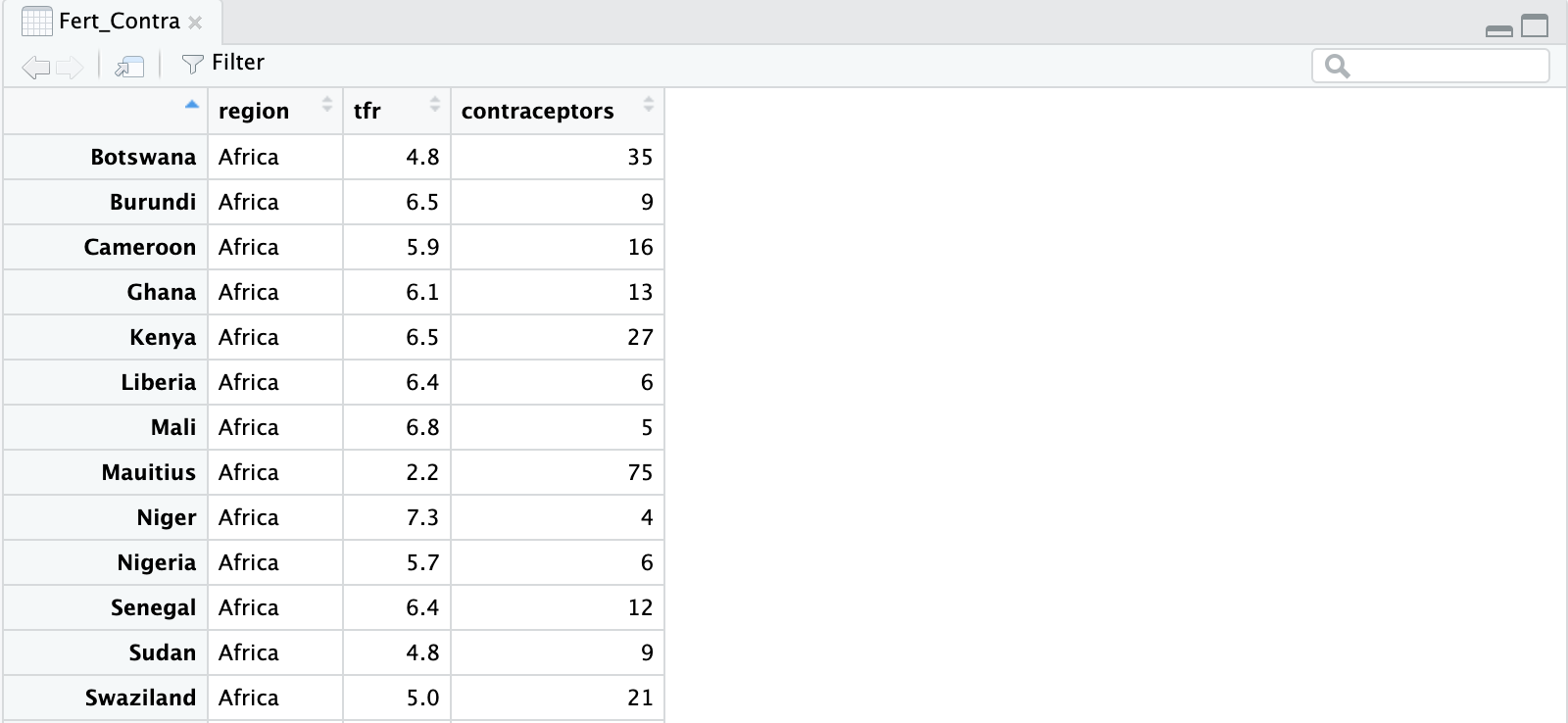note

You also need to load the ggplot package into your workspace before you can use it. To do so, type `library(ggplot2)` into the console.

## What are aesthetics?​

Aesthetics are used to map different information to certain visual properties in a plot. Mapping means that a variable from the specified dataset is linked to the plot in R to display it accurately. Examples of aesthetics include the size, colour or shape of the points in a plot. The mapping of variables to the x- or y-axis are also aesthetics.

You apply the aesthetic function by using `aes()` after typing `ggplot2`. Consider the following:

``# create a scatter plot displaying the relationship between the total fertility rate ggplot(Fert_Contra, aes(x = tfr, y = contraceptors)) + geom_point()``

This example of code contains the three essential parts that are always needed to create a graph in ggplot2:

• `ggplot()`: the coordinate system that you add layers to. The `Fert_Contra` argument is the dataset that you want to visualize.
• `aes(x = tfr, y = contraceptors)`: The aesthetics argument, that maps the variables to the x and y axes of the plot.
• `+ geom_point()`: adds a geometry layer to your plot. This particular geometry function adds a layer of points so that a scatter plot is created. There are different geometry functions that create different plots (which will be explained in the next chapter).

The resulting plot will look like this: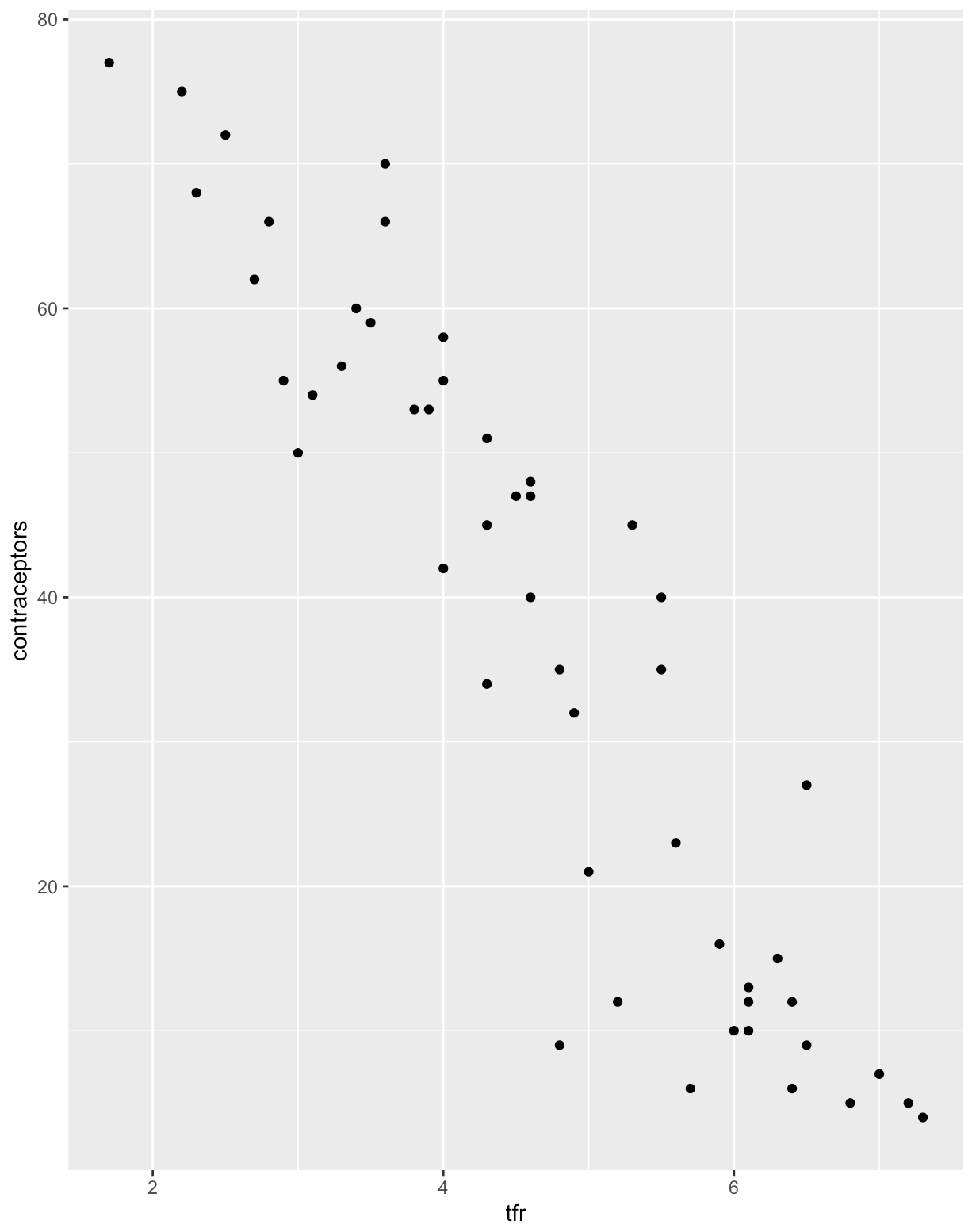You will usually have more variables in your dataset that you may want to display on a single plot. You can display more variables in one plot by mapping them to additional aesthetics such as colour and size.

### Colour Aesthetics​

To use this aesthetic add `colour =` inside the `aes()` function:

``# create a plot that shows the relationship between tfr and contraceptors amongst different regions ggplot(Fert_Contra, aes(x= tfr, y = contraceptors, colour = region))     + geom_point()``

In the above code we added an argument in the `aes()` function in which the region variable was mapped to the `colour` aesthetics. This assigns a unique colour to each value in the variable. ggplot2 automatically adds a legend to the plot to show which values correspond to which colours: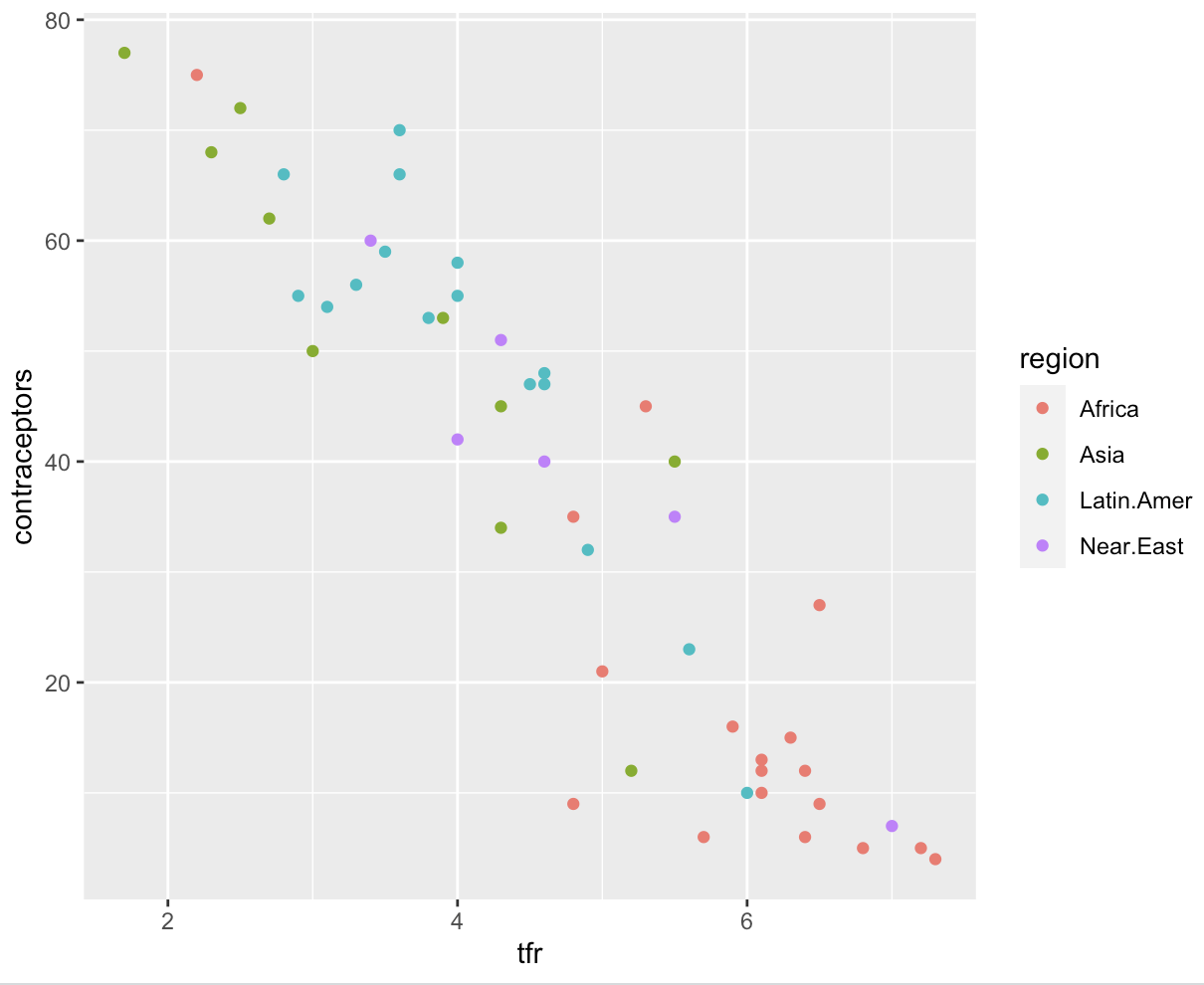### Size Aesthetics​

If you have a variable with numerical data, you can use `size`. To use this aesthetic, you add `size =` inside the `aes()` function:

``# create a plot that shows the relationship between tfr and contraceptors amongst different regions ggplot(Fert_Contra, aes(x= tfr, y = contraceptors, size = region)) + geom_point()``

In this example, `region` was mapped to the size aesthetic, where the size of each point corresponds to a certain region. However, since mapping a categorical data with the size aesthetic is not recommended, R will send out a warning along the lines of "Using size for a discrete variable is not advised":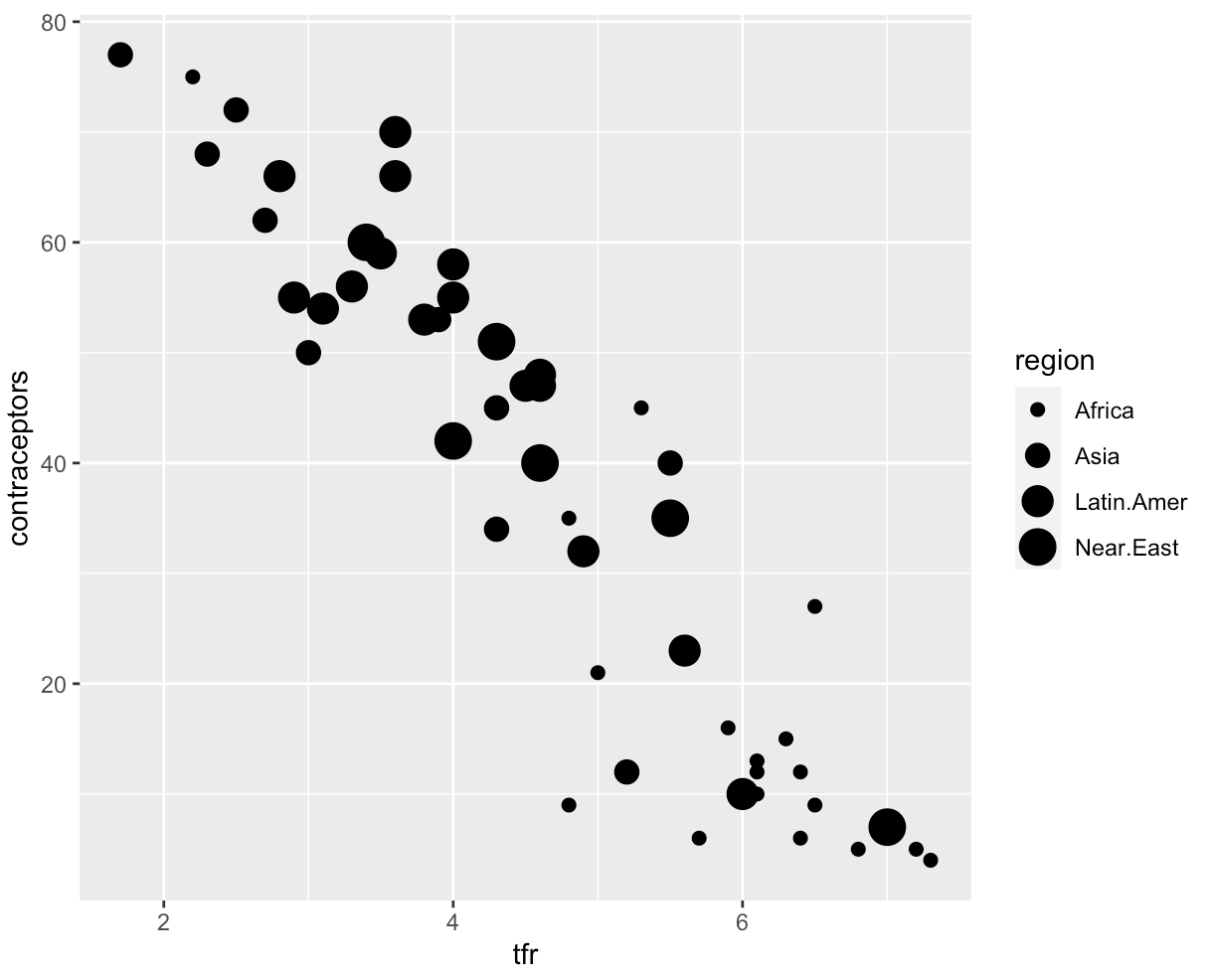note

The greater the size of the dots, the larger the value the dot represents!

### Alpha Aesthetics​

Using the `alpha` argument you can alter the transparency of your points:

``# create a plot that shows the relationship between tfr and contraceptors amongst different regions ggplot(Fert_Contra, aes(x= tfr, y = contraceptors, alpha = region)) + geom_point()``

In this case, `region` was mapped to the `alpha` aesthetic, with the transparency corresponding with a region. There will also be a warning message since we are mapping a categorical data with an aesthetic that is better used for numerical data: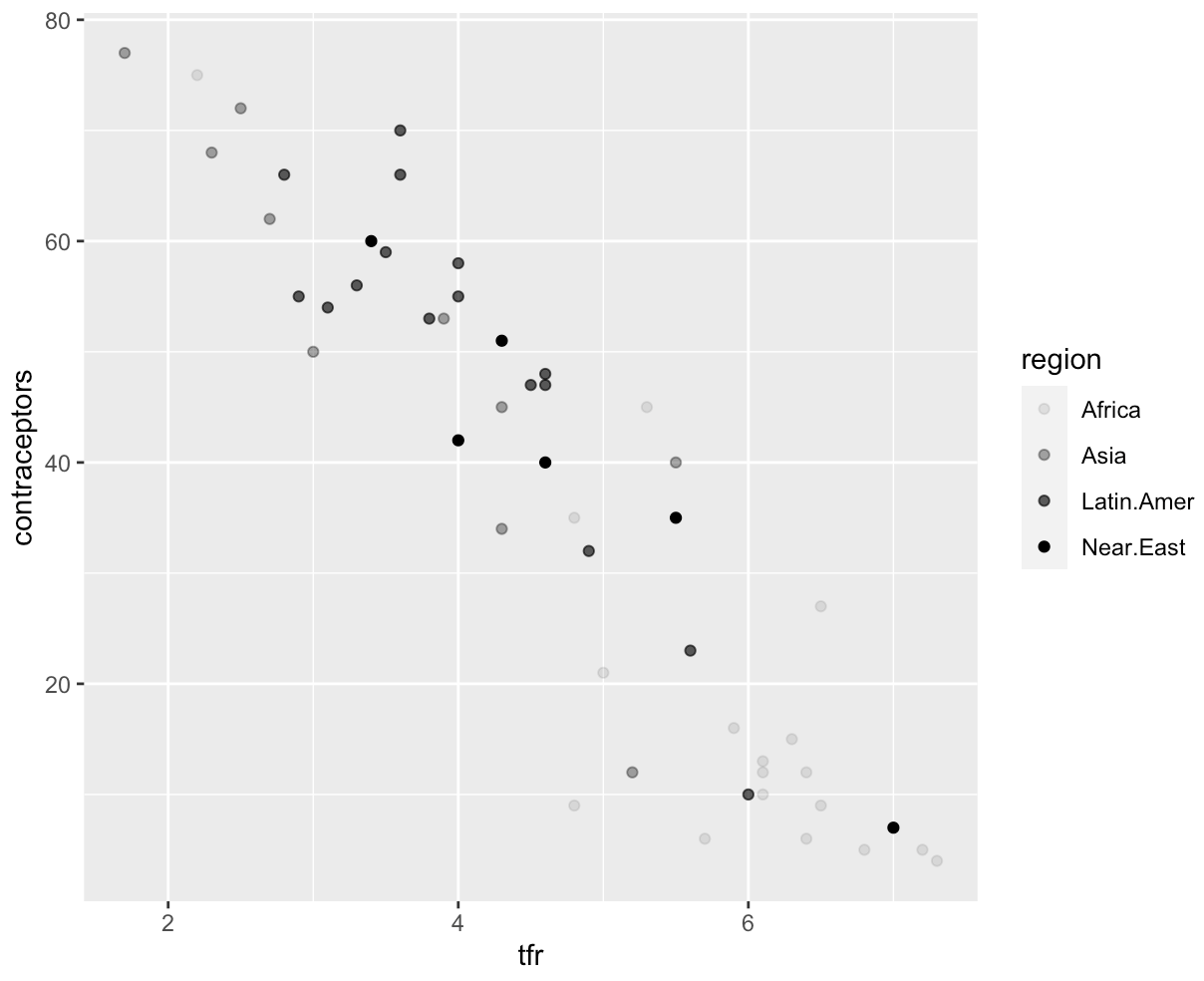info

The alpha argument can also be used to change the transparency of all points in a plot to the same value. The default value for alpha is 1. To reduce the transparency, you can set `alpha` to a value less than 1 (and larger than 0), outside `aes()` and inside the geom layer:

``ggplot(Fert_Contra, aes(x= tfr, y = contraceptors)) + geom_point(alpha = 0.7)``

Which gives the following result: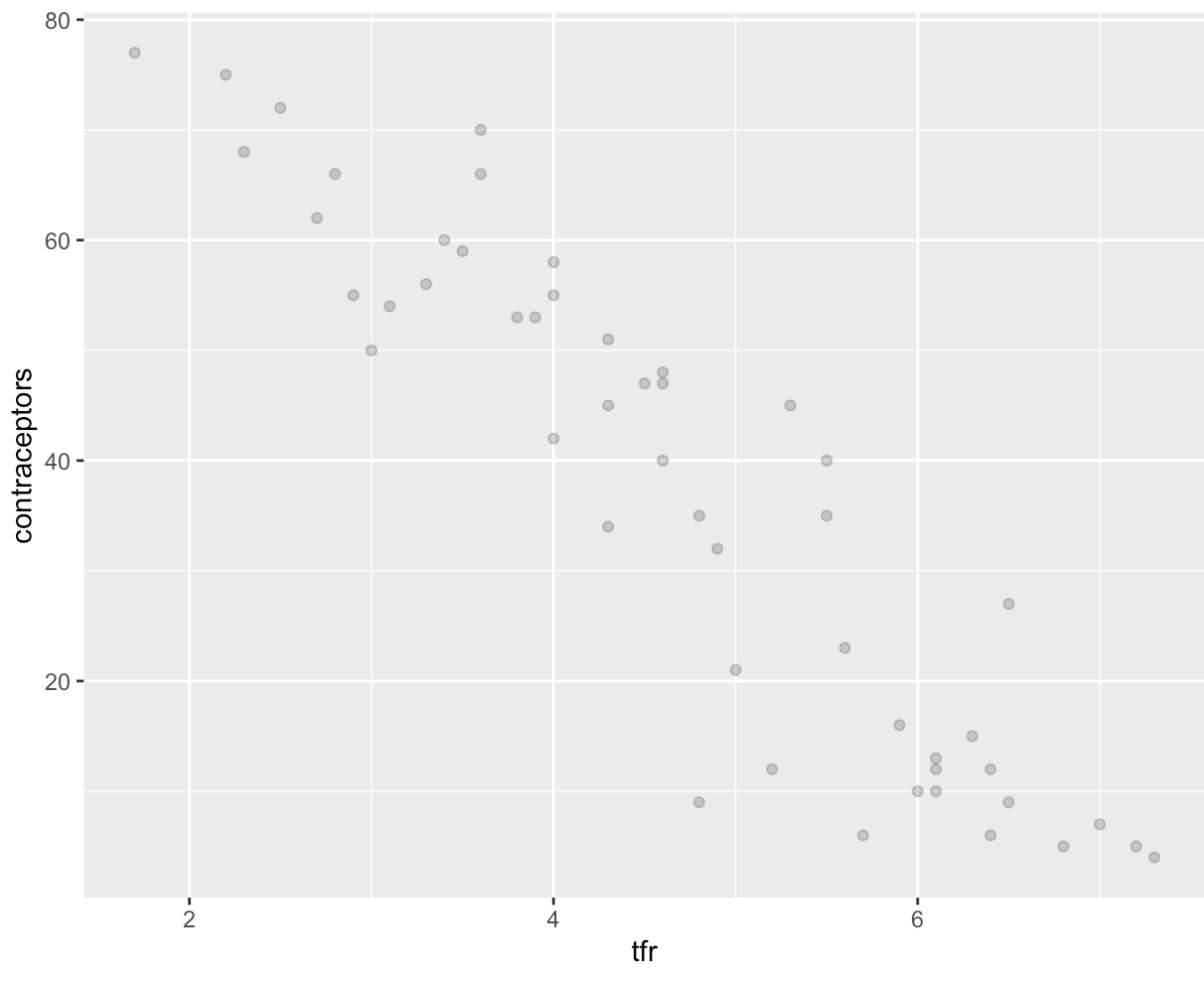This syntax is usually used in an attempt to solve the problem of overplotting; in large datasets the dots are often all over each other and cannot be distinguished!

### Shape Aesthetics​

Categorical variables can also be mapped onto a plot using the shape aesthetic:

``# create a plot that shows the relationship between tfr and contraceptors amongst different regions ggplot(Fert_Contra, aes(x= tfr, y = contraceptors, shape = region)) + geom_point()``

This will translate into the following plot: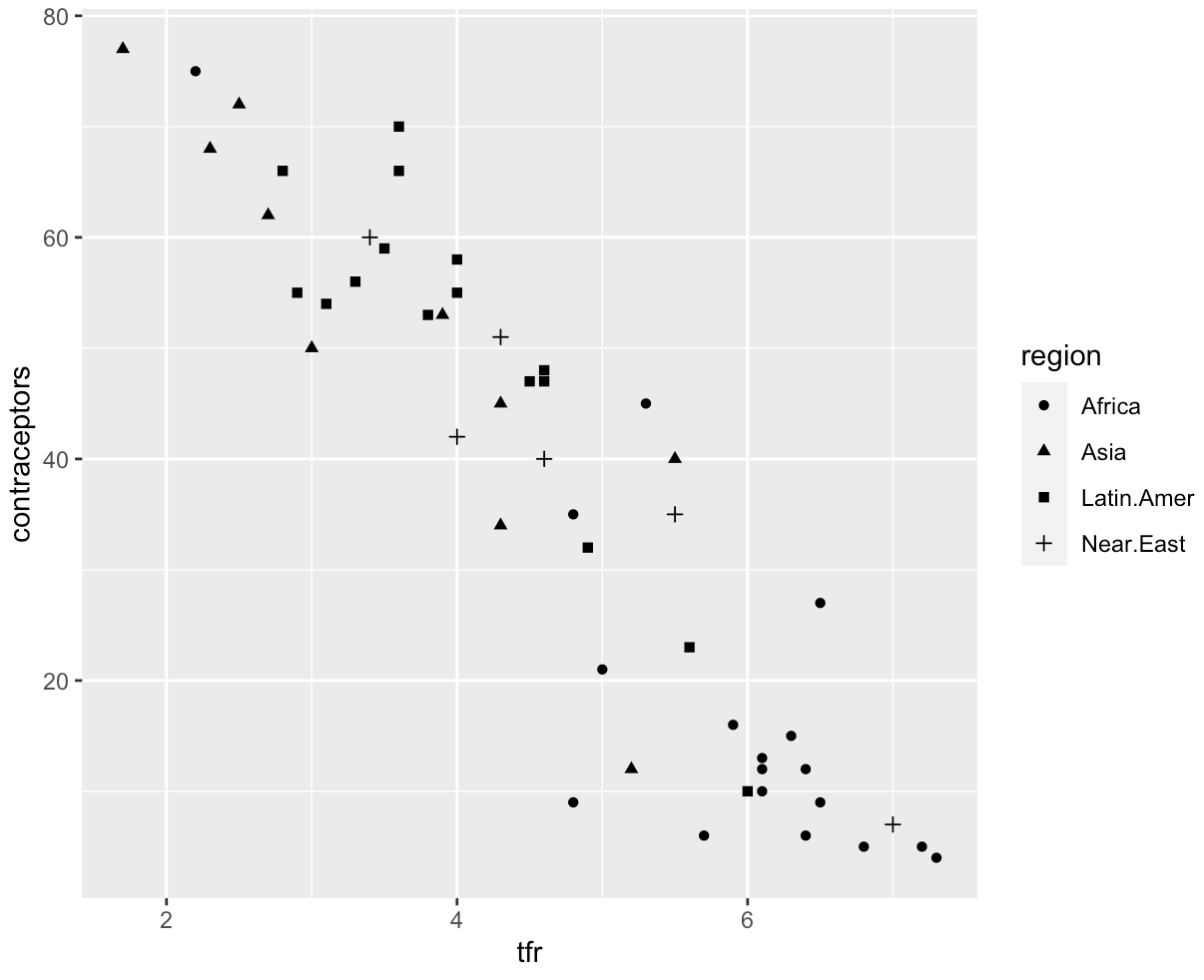ggplot2 has 25 different shapes to choose from. The shapes seem to repeat themselves, but there are differences in their properties:

• Shapes 0 to 14 can only change the colour of their outline using the `colour` argument.
• Shapes 15 to 18 are filled with `colour`.
• Shapes 21 to 24 have an outline which can be modified using `colour` and the insides can be modified using `fill`.

## Common aesthetics summary​

The table below shows a summary of many aesthetics that are used to map information to a plot:

Aesthetic:What it does:
`x`maps variables to the x-axis
`y`maps variables to the y-axis
`colour`maps variables (preferably categorical) to outline colour
`fill`maps variables (preferably categorical) to fill colour
`shape`maps variables (preferably categorical) to shape
`size`maps variables (preferably numerical) to size
`alpha`maps variables (preferably numerical) to transparency
`line type`maps variables to line type
`labels`maps variables to certain words.